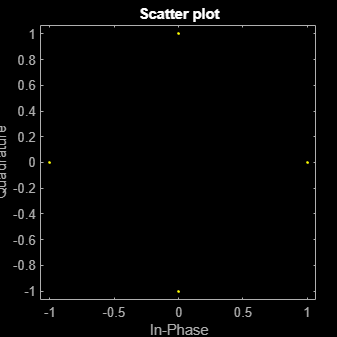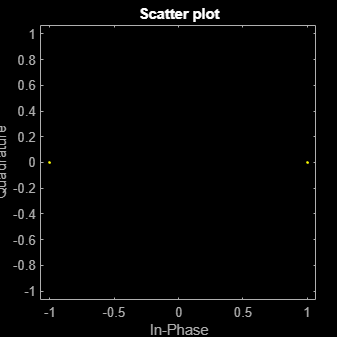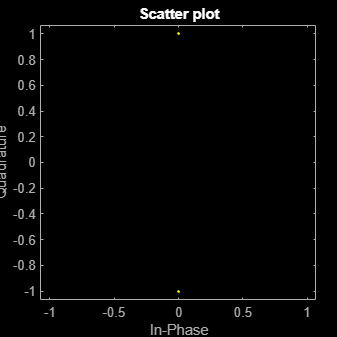Main Content

# wlanConstellationMap

Constellation mapping

## Syntax

``y = wlanConstellationMap(bits,numBPSCS) ``
``y = wlanConstellationMap(bits,numBPSCS,phase) ``

## Description

example

````y = wlanConstellationMap(bits,numBPSCS) `maps the input sequence `bits` using the number of coded bits per subcarrier per spatial stream, `numBPSCS`, to one of the following modulations: BPSK, QPSK, 16QAM, or 64QAM, as per IEEE® 802.11™-2012, Section 18.3.5.8256QAM, as per IEEE 802.11ac™-2012, Section 22.3.10.9.11024QAM, as per IEEE 802.11-16/0922r2 The constellation mapping is performed column-wise.```

example

````y = wlanConstellationMap(bits,numBPSCS,phase) `rotates the constellation points counterclockwise by the number of radians specified in `phase`.```

## Examples

collapse all

Perform a 256QAM mapping, as defined in IEEE® 802.11ac™-2013 Section 22.3.10.9.1.

Create the sequence of data bits.

`bits = randi([0 1],416,1,'int8');`

Perform the constellation mapping on the data bits with a 256QAM modulation.

```numBPSCS = 8; mappedData = wlanConstellationMap(bits,numBPSCS);```

The size of the output returned by this modulation equals the size of the input sequence divided by eight.

`size(mappedData)`
```ans = 1×2 52 1 ```

Perform a $\frac{\pi }{2}$-BPSK mapping on a sequence of data bits as defined in IEEE® 802.11ad™-2012 Section 21.6.3.2.4.

Create the sequence of data bits.

`bits = randi([0 1],512,1);`

Perform the BPSK mapping on the data bits with a rotation of $\frac{\pi }{2}$ radians. Note that the size of the constellation rotation `phase` is equal to the size of input sequence.

```numBPSCS = 1; phase = pi*(0:size(bits,1)/numBPSCS-1).'/2; mappedData = wlanConstellationMap(bits,numBPSCS,phase);```

As we performed a BPSK mapping, the number of symbols per bit is one, therefore the size of the output is equal to the size of the original sequence.

`size(mappedData)`
```ans = 1×2 512 1 ```

Display the modulated signal constellation using the `scatterplot `function.

`scatterplot(mappedData);`Perform BPSK and QBPSK demapping for different OFDM symbols for the VHT-SIG-A field by using a soft demodulation. The mapping is defined in IEEE® 802.11ac™-2013 Section 22.3.8.3.3 for the VHT-SIG-A field.

Create the sequence of data bits. Place the two OFDM symbols in columns.

`bits = randi([0 1],48,2,'int8');`

Perform constellation mapping on the data bits. Specify the size of constellation rotation `phase` as the number of columns in the input sequence. The first column is mapped with a BPSK modulation. The second column is modulated with a QBPSK modulation.

```numBPSCS = 1; phase = [0 pi/2]; mappedData = wlanConstellationMap(bits,numBPSCS,phase);```

Display the modulated signal constellation by using the `scatterplot `function. The first plot shows the data after the BPSK modulation, and the second plot shows the QBPSK-modulated symbols.

`scatterplot(mappedData(:,1))``scatterplot(mappedData(:,2))`## Input Arguments

collapse all

Input sequence of bits to map into symbols, specified as a binary vector, matrix, or multidimensional array.

Data Types: `double` | `int8`

Number of coded bits per subcarrier per spatial stream, specified as log2(M), where M is the modulation order. Therefore, `numBPSCS` must equal:

• 1 for a BPSK modulation

• 2 for a QPSK modulation

• 4 for a 16QAM modulation

• 6 for a 64QAM modulation

• 8 for a 256QAM modulation

• 10 for a 1024QAM modulation

Example: `4`

Data Types: `double`

Constellation rotation in radians, specified as a scalar, vector, or multidimensional array. The size of `phase` must be compatible with the size of the input `bits`. `phase` and `bits` have compatible sizes if, for each corresponding dimension, the dimension sizes are either equal or one of them is 1. When one of the dimensions of `bits` is equal to 1, and the corresponding dimension of `phase` is larger than 1, then the output dimensions have the same size as the dimensions of `phase`.

Example: `pi*(0:size(bits,1)/numBPSCS-1).'/2;`

Data Types: `double`

## Output Arguments

collapse all

Mapped symbols, returned as a complex vector, matrix, or multidimensional array. `y` has the same size as `bits`, except for the number of rows, which is equal to the number of rows of `bits` divided by `numBPSCS`.

## See Also

Introduced in R2017b

## SupportGet trial now